Full Text:<2968>

Summary:<1667>

CLC number: X703

On-line Access: 2014-05-04

Revision Accepted: 2014-02-17

Crosschecked: 2014-04-25

Cited: 3

Clicked: 5586

Citations:  Bibtex RefMan EndNote GB/T7714

 Journal of Zhejiang University SCIENCE A 2014 Vol.15 No.5 P.374-384 http://doi.org/10.1631/jzus.A1300262Applying process analytical technology framework to optimize multiple responses in wastewater treatment process

 Author(s):  Abbas Al-Refaie Affiliation(s):  . Department of Industrial Engineering, The University of Jordan, Amman 11942, Jordan Corresponding email(s):   abbas.alrefai@ju.edu.jo Key Words:  Fuzzy goal optimization, Multiple responses, Wastewater, Process analytical technology (PAT) Share this article to： More <<< Previous Article|

Abbas Al-Refaie. Applying process analytical technology framework to optimize multiple responses in wastewater treatment process[J]. Journal of Zhejiang University Science A, 2014, 15(5): 374-384.

@article{title="Applying process analytical technology framework to optimize multiple responses in wastewater treatment process",
author="Abbas Al-Refaie",
journal="Journal of Zhejiang University Science A",
volume="15",
number="5",
pages="374-384",
year="2014",
publisher="Zhejiang University Press & Springer",
doi="10.1631/jzus.A1300262"
}

%0 Journal Article
%T Applying process analytical technology framework to optimize multiple responses in wastewater treatment process
%A Abbas Al-Refaie
%J Journal of Zhejiang University SCIENCE A
%V 15
%N 5
%P 374-384
%@ 1673-565X
%D 2014
%I Zhejiang University Press & Springer
%DOI 10.1631/jzus.A1300262

TY - JOUR
T1 - Applying process analytical technology framework to optimize multiple responses in wastewater treatment process
A1 - Abbas Al-Refaie
J0 - Journal of Zhejiang University Science A
VL - 15
IS - 5
SP - 374
EP - 384
%@ 1673-565X
Y1 - 2014
PB - Zhejiang University Press & Springer
ER -
DOI - 10.1631/jzus.A1300262

Abstract:
In this research, the process analytical technology (PAT) framework is used to optimize the performance of the wastewater treatment process in poultry industry. Two responses, turbidity and sludge volume index (SVI), are of main manufacturer’s interest. Initially, the moving average (MA) and moving range (MR) control charts are established for each response. The 33 full factorial design with two replicates is then used for conducting experimental work. The weighted additive model in fuzzy goal programming is formulated, and then employed to determine the combination of optimal factor settings. Finally, confirmation experiments follow at the combination of optimal factor settings. The results show that the actual process index for turbidity is improved from 1.34 to 5.5, while it is enhanced from 1.46 to 1.93 for SVI. Moreover, the multiple process capability index is improved significantly from 1.95 to 10.6, which also indicates that the treatment process becomes highly capable with both responses concurrently. Further, the process standard deviations at initial (optimal) factor settings are 2.16 (1.27) and 6.02 (3.39) for turbidity and SVI, respectively. These values show significant variability reductions in turbidity and SVI by 41.22% and 77.75%, respectively. Such improvements will lead to huge savings in quality and productivity costs. In conclusion, the PAT framework is found to be an effective approach for optimizing the performance of the wastewater treatment process with multiple responses.

## 1.  Introduction

In recent years, there has been an increased interest in wastewater treatment for environmental conservation. As a consequence, the industrial sector is obligated to treat its own waste and lower its organic load to a level that the ecosystem can cope with. The efficient employment of engineering concepts in parallel with process knowledge can lead to tangible improvements in process capability and potential.

The industrial wastewater treatment process can be defined as chemical, biological and/or mechanical procedures applied to industrial discharge in order to increase the quality of wastewater by reducing its organic and inorganic pollutants. The treated water quality can be described by several physical parameters, such as turbidity and sludge volume index (SVI), which are considered as vital quality responses that have large effects on effluent water quality.

Process analytical technology (PAT) is a system that analyses and controls manufacturing processes based on timely measurements of critical quality characteristics and performance attributes of raw materials and in-process products to ensure acceptable end-product quality (CDER, ). The PAT framework is a combination of tools that, when used within a system, can provide useful means for acquiring information resulting in continual process improvement, such as multivariate techniques, data acquisition and analysis, process analysis methods, process control tools, and continual improvement and a knowledge management approach. A summary of the tools that are commonly used in the PAT framework is displayed in Fig. 1.

Recently, improving the performance of the wastewater treatment process has received significant research attention. Wang et al. () used response surface methodology to optimize the coagulation-flocculation process for paper-recycling wastewater treatment. Wang et al. () applied a uniform design in parallel with response surface methodology to optimize the coagulation-flocculation process for pulp mill wastewater treatment. Furthermore, several approaches have been proposed for optimizing process performance with multiple responses (Al-Refaie et al., ; ; Al-Refaie, ; ; ; ; Al-Refaie and Al-Tahat, ; Muhammad et al., ; Salmasnia et al., ). Among the efficient optimization approaches is the weighted additive model in fuzzy goal programming, which considers the preferences for quality responses when determining the combination of optimal process factor’s settings. The weighted additive model has been applied to optimize process performance in several industrial applications (Yaghoobi et al., ; Yücel and Guneri, ; Al-Refaie and Li, ; Al-Refaie et al., ). This research aims at applying the PAT framework, including the weighted additive model, to optimize the performance of the coagulation-flocculation process in wastewater treatment.

## 2.  PAT framework

The PAT framework was implemented to improve the performance of the wastewater treatment process and is described as follows.

### 2.1.  Identifying critical process attributes

The wastewater treatment process is mapped as shown in Fig. 2. The inflow stream is screened at first using two rotary screens to remove coarse materials that could damage the subsequent process equipment. The screened flow is then physically treated in a dissolved air flotation unit (DAF) where it is exposed to a pressurized air stream in order to float its oil and fat content. The floating fat layer is then scraped off by a skimming device. The treated stream is passed through an aeration process using a selector tank to enhance the growth of floc-forming bacteria instead of filamentous bacteria to provide an activated sludge with better settling and thickening properties. The aerated stream is then biologically treated in a treatment tank where the dissolved and particulate constituents are biologically degraded. The last treatment process is coagulation-flocculation in which sludge is separated from water by adding certain coagulant and flocculant agents. The flocculated sludge will float by means of a pressurized air stream where it is scraped by a skimming device. On the other hand, the treated water is then chlorinated and stored, to be used for irrigation purposes.

### 2.2.  Real-time monitoring

A control chart plots the average of measured values of quality responses in samples taken from the process versus the sample number. The chart has a center line (CL) as well as upper and lower control limits (UCL and LCL, respectively). The CL represents where this process characteristic should fall if no assignable causes for variability are present. The moving average (MA) and moving range (MR) control charts are found to be effective ones in detecting small process shifts and process variability, respectively. The turbidity is measured using a portable DR/890 colorimeter instrument, while the SVI is measured manually using the standard method. The MA and MR control charts for turbidity and SVI are constructed and depicted in Figs. 3 and 4, respectively. In Fig. 3 for turbidity, the UCL, CL, and LCL of the MA control chart are calculated and found respectively equal to 24.81, 20.22, and 15.64 NTU (nephelometric turbidity units), while the corresponding parameter values in the MR control chart are equal to 7.964, 2.438, and 0 NTU, respectively. In MA and MR control charts, neither point falls outside the control limits nor does a significant pattern exist in the charts. Hence, it is concluded that the process is in control for turbidity and SVI. Furthermore, the corresponding lower limit (LSL) and upper specification limit (USL) for the turbidity are 0 and 30 NTU, respectively, while the smaller SVI value is preferred within the specification range. Observing the CL (=20.22 NTU) of the MA control chart for turbidity, it is noted that the turbidity is significantly larger than the above maximum allowable target value of 15 NTU. From Fig. 4 for SVI, the UCL, CL, and LCL of the MA (MR) control chart are estimated respectively as 92.69 (22.19), 79.92 (6.79), and 67.15 (0) ml/g. The LSL and USL of SVI are 50 and 100 ml/g, respectively, where it is preferred at the nominal value of 70 ml/g. Hence, the SVI is considered the nominal best type response. It is found that the CL (=79.92 ml/g) for SVI is shifted from the nominal value. Consequently, improvement actions are required to improve process performance.Fig.3
MA chart (a) and MR chart (b) for turbidity at initial factor settings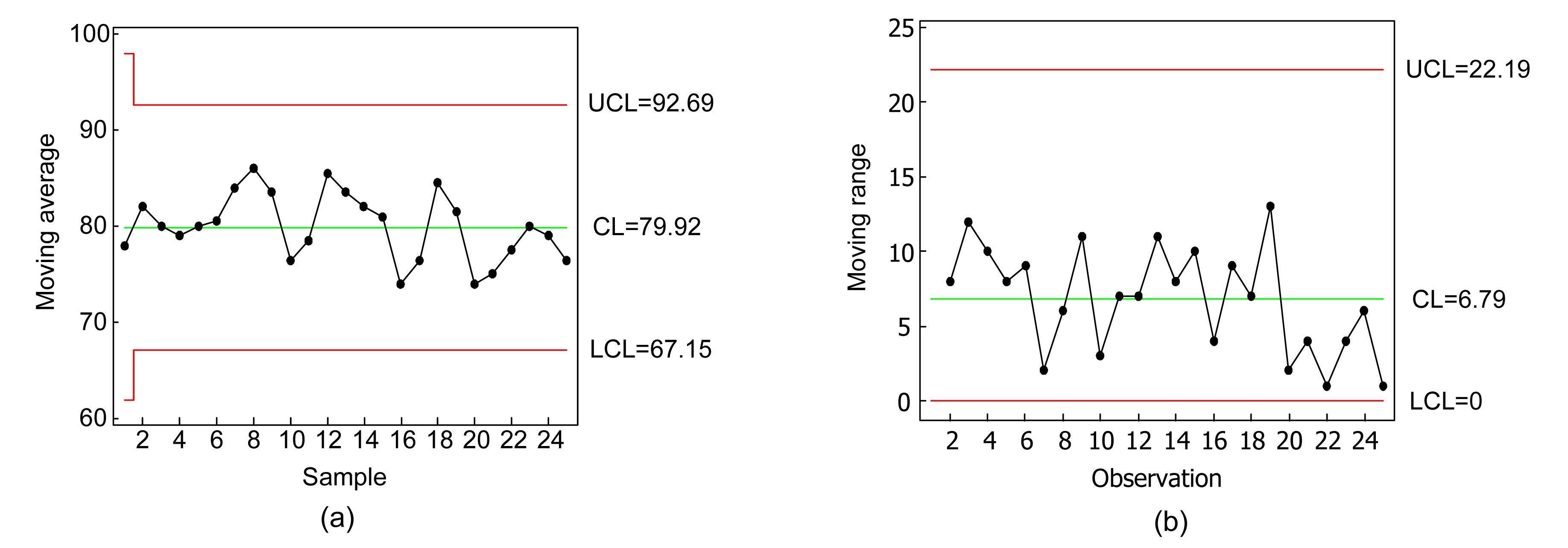Fig.4
MA chart (a) and MR chart (b) for SVI at initial factor settings

### 2.3.  Modeling and prediction

In the third step of the PAT framework, a mathematical relationship is developed for both turbidity, y1, and SVI, y2, with the critical process factors. Based on process knowledge, the main controllable process factors thought to affect the wastewater quality characteristics are flocculant dose (x1, mg/L), coagulant dose (x 2, mg/L), and pH (x 3). The current process factor settings for the wastewater treatment process are: flocculant dose=8 mg/L, coagulant dose=50 mg/L and pH=6. Each factor is assigned at three levels: 1: low, 2: middle, and 3: high. The physical values for factor levels are listed in Table 1.

#### Table 1

Physical values for factor levels
 Level Factor x 1 x 2 x 3 1 6 40 4 2 12 60 6 3 18 80 8

For the present process, 33 full factorial design with two replicates I and II, enables investigation of three three-level factors with their interactions without the need to ignore the three-way interaction. The designed experiment is conducted and tested using jar test apparatus which is specially designed to be used with conventional wastewater treatment processes such as coagulation, flocculation, and sedimentation. Turbidity and SVI are measured once in each replicate. Table 2 displays the experimental results for both quality responses.

#### Table 2

Experimental results for both quality responses
 Exp. No. Process factor Turbidity (y 1) replicate SVI (y 2) replicate x 1 x 2 x 3 I II I II 1 6 40 4 9.3 9.2 89.2 90.1 2 6 40 6 25.7 24.0 101.6 102.2 3 6 40 8 23.2 24.6 103.2 105.3 4 6 60 4 20.8 20.1 107.9 108.2 5 6 60 6 29.1 28.7 106.7 107.5 6 6 60 8 26.5 28.2 121.3 123.4 7 6 80 4 45.3 46.4 122.4 121.8 8 6 80 6 36.6 35.2 133.6 132.8 9 6 80 8 40.2 39.7 136.9 137.1 10 12 40 4 4.5 4.2 78.8 77.2 11 12 40 6 10.1 9.8 98.6 96.4 12 12 40 8 13.3 12.9 104.3 103.9 13 12 60 4 14.5 14.3 106.0 104.2 14 12 60 6 19.1 18.3 103.1 101.3 15 12 60 8 16.6 17.2 101.9 105.2 16 12 80 4 20.2 21.8 115.0 118.1 17 12 80 6 30.2 29.4 124.1 119.2 18 12 80 8 25.4 24.3 126.3 129.8 19 18 40 4 8.2 8.9 76.1 79.8 20 18 40 6 9.5 10.2 73.3 75.2 21 18 40 8 10.3 9.6 83.8 87.9 22 18 60 4 8.1 7.7 79.2 79.4 23 18 60 6 10.7 10.2 92.4 92.6 24 18 60 8 12.2 12.6 101.1 100.6 25 18 80 4 15.8 15.2 95.8 103.5 26 18 80 6 16.3 15.5 101.6 105.1 27 18 80 8 17.8 17.3 115.7 116.3

The weighted additive model will be employed to obtain the optimal values of process factor settings while considering the preferences of process/product engineers as follows.

Step 1: Estimate the relationship between each of y 1 and y 2 responses and the process factors x 1, x 2, and x 3 using statistical multiple linear regression as follows: where f is assumed to be a linear function, yk denotes the kth quality response, and xj represents the jth process factor, j=1, 2, …, J.

Analyses of variance (ANOVA) for the experimental values of y 1 and y 2 are conducted to determine significant main and interaction effects. ANOVA results for both responses are displayed in Table 3. Obviously, all main two-way interactions and three-way interactions are found to be significant for both responses. Moreover, the corresponding significance tests of linear regression coefficients are listed in Table 4.

#### Table 3

Analysis of variance for turbidity
 Term Degrees of freedom Turbidity (y 1) SVI (y 2) Sum of squares Mean sum of squares F P Sum of squares Mean sum of squares F P x 1 2 2571.40 1285.70 3321.91 0.000 4389.11 2194.56 627.35 0.000 x 2 2 2027.67 1013.83 2619.48 0.000 7837.79 3919.40 1120.42 0.000 x 3 2 212.80 106.41 274.94 0.000 1758.74 879.37 251.38 0.000 x 1 x 2 4 348.00 87.00 224.78 0.000 51.54 12.89 3.68 0.016 x 1 x 3 4 38.68 9.67 24.98 0.000 61.09 15.27 4.37 0.007 x 2 x 3 4 133.17 33.29 86.02 0.000 64.19 16.05 4.59 0.006 x 1 x 2 x 3 8 304.93 38.12 98.48 0.000 678.18 84.77 24.23 0.000 Error 27 10.45 0.39 94.45 3.50 Total 53 5647.12 14936.09 * R 2=99.81%, R 2 (adjusted)=99.64% R 2=99.37%, R 2 (adjusted)=98.76%

• * R 2: coefficient of determination

• #### Table 4

Results of test of significance for factor coefficients
 Predictor Turbidity (y 1) SVI (y 2) Coefficient Standard Error T P Coefficient Standard Error T P Constant −84.59 18.03 −4.69 0.000 31.46 25.58 1.23 0.225 x 1 4.718 1.391 3.39 0.001 0.279 1.974 0.14 0.888 x 2 1.8324 0.2900 6.32 0.000 1.2419 0.4114 3.02 0.004 x 3 12.767 2.900 4.40 0.000 7.111 4.114 1.73 0.091 x 1 x 2 −0.09618 0.02237 −4.30 0.000 −0.03627 0.03174 −1.14 0.259 x 2 x 3 −0.18417 0.04664 −3.95 0.000 −0.06312 0.06616 −0.95 0.345 x 1 x 3 −0.7160 0.2237 −3.20 0.002 −0.2408 0.3174 −0.76 0.452 x 1 x 2 x 3 0.011042 0.003598 3.07 0.004 0.004245 0.005105 0.83 0.410 * R 2=90.3%, R 2 (adjusted)=88.80% R 2 =92.6%, R 2 (adjusted)=91.50%

• * R 2: coefficient of determination

•  Finally, the normality tests for turbidity and SVI are constructed in Figs. 5 and 6, respectively. Obviously, in the plots of fitted values versus the normalized residual and the normal probability plots for residuals, there is no obviously significant pattern or outliers or a relationship between the fitted values and their corresponding residual values. Consequently, mathematical relationships for y 1 and y 2 are expressed in Eqs. (2) and (3), respectively. , .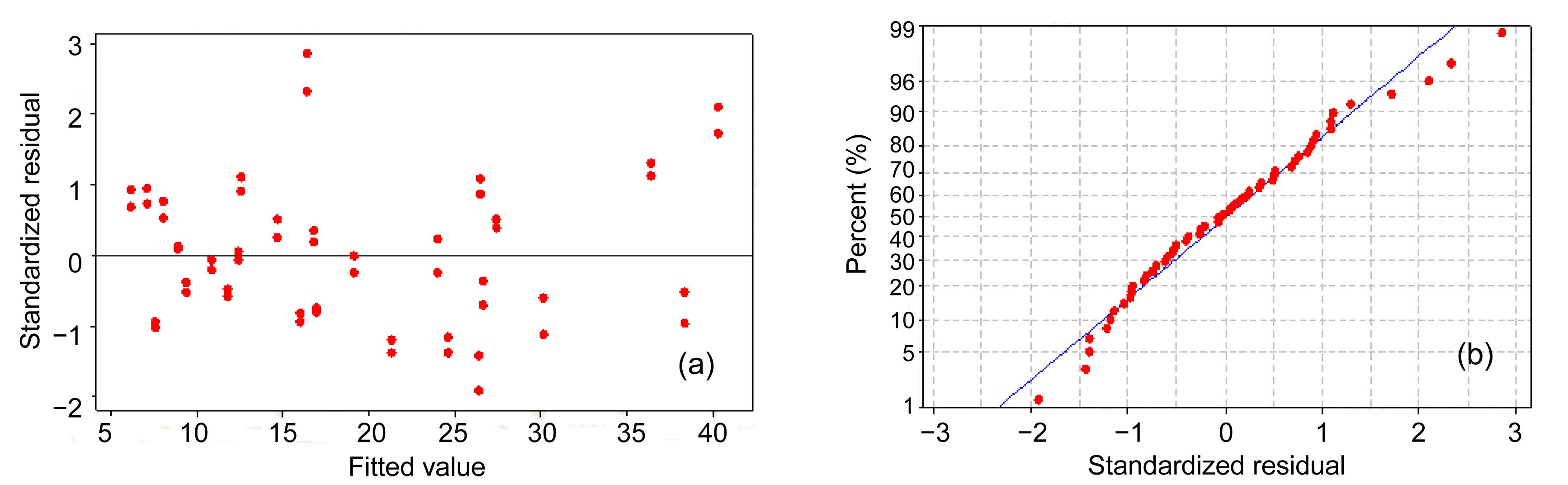Fig.5
Normality test results for turbidity (y 1)
(a) Residual versus the fitted value; (b) Normal probability plot of the residuals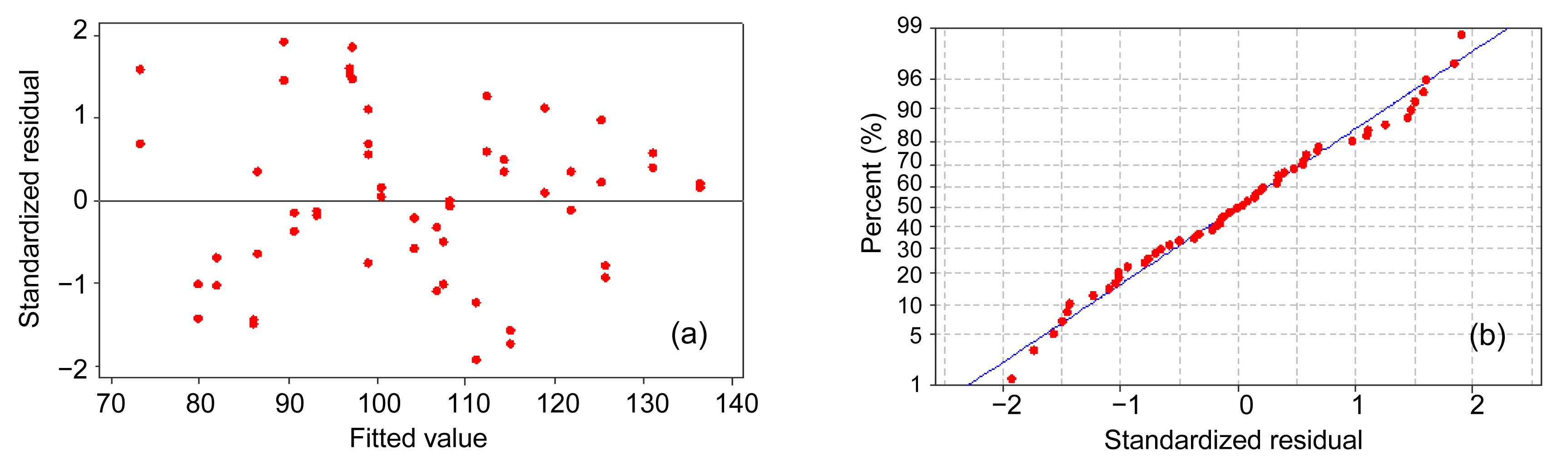Fig.6
Normality test results for SVI (y 2)
(a) Residual versus the fitted value; (b) Normal probability plot of the residuals

Step 2: Select proper membership function (MF) for y 1, y 2, and xj . Let the MFs for turbidity and SVI be denoted as and , respectively, which are decided as follows.

1. For the turbidity, y 1, the suitable is depicted in Fig. 7a and is defined as where is the imprecise fuzzy value of y 1 specified by process engineers, and is the maximal positive admissible violation from . The corresponding goal constraints are given by where denotes the positive deviation of y 1 from . Based on the engineers’ preferences, the values of and are decided to be 15 NTU, while the maximal acceptable value of y 1 is 30 NTU. Consequently, and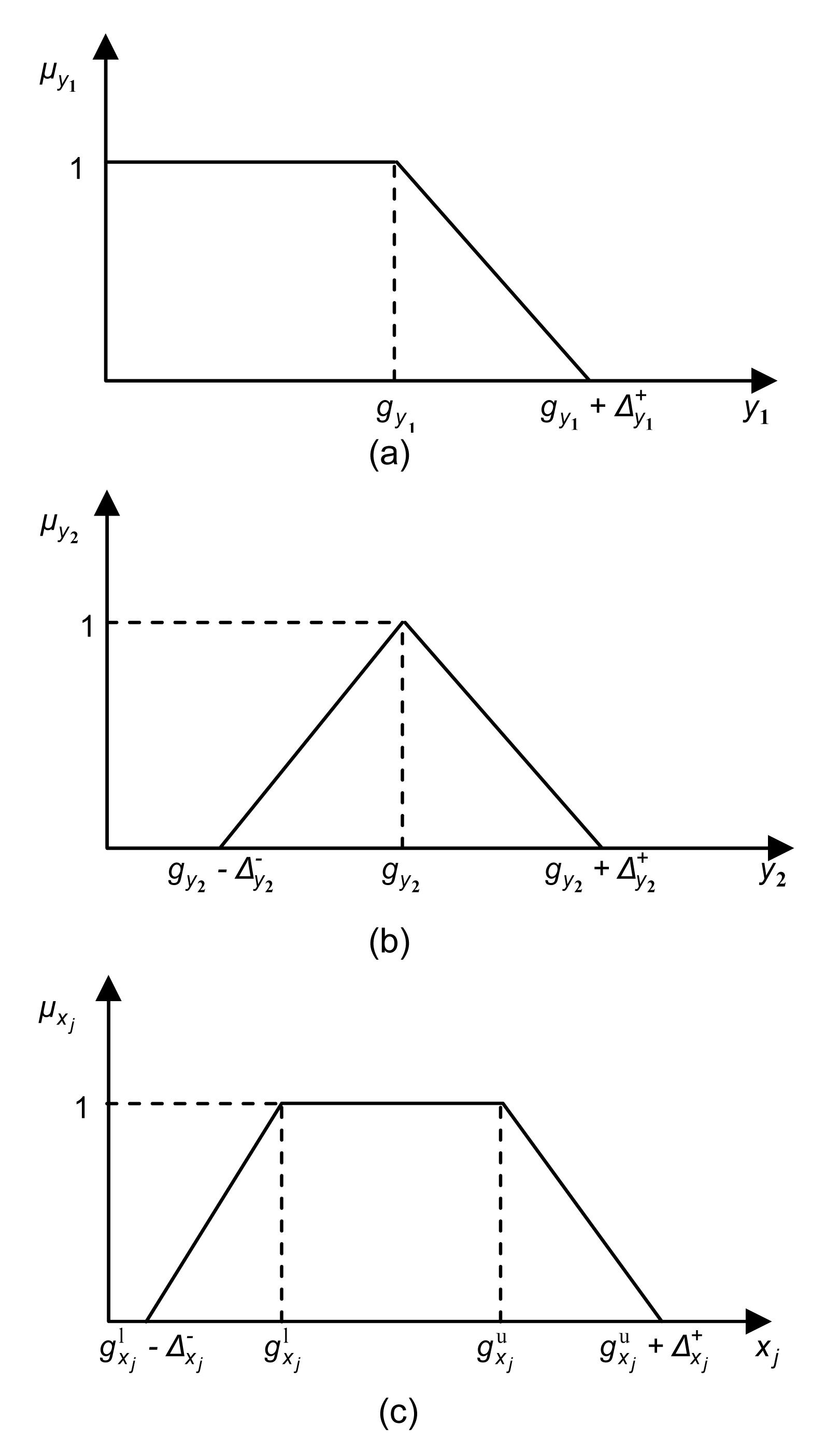Fig.7
MFs for responses and process factors
(a) MF for y 1; (b) MF for y 2; (c) MF for xj

2. Similarly, the suitable MF, , for SVI is shown in Fig. 7b, or mathematically where is the target of y 2. and denote the maximal negative and positive admissible violations from , respectively. The corresponding goal constraints are expressed as where and represent the negative and positive deviations from , respectively. The process engineer decided that the values of , , and are 60, 20, and 40 ml/g, respectively. The minimal and maximal acceptable values of y 2 are 50 and 100 ml/g. Then, and

3. Similarly, there is no information on the exact target of x 1, x 2, and x 3. Then, the suitable MF, , is described in Fig. 7c and is defined as where and are the lower and the upper limits of xj , respectively. and are the maximal negative and positive admissible violations from and , respectively. The corresponding constraints are then formulated as where and represent the negative and positive deviations from and , respectively. It is decided that the values of and equal 0.1. For example, is defined as

The corresponding constraints are defined as

The MFs with their corresponding constraints for x 1 and x 2 are formulated in a similar manner.

Step 3: The objective function of the weighted additive GP model is to minimize the sum of the weighted deviations of responses and process variables.

Accordingly, the objective function is to minimize .

Substituting the values of deviations weights, Eq. (10) is formulated as .

Step 4: A linear programming model is formulated using steps 1–4 as follows:

Minimize Z subject to:

Solving the model, the obtained optimal process conditions are: flocculant dose, x 1, of 18 mg/L, coagulant dose, x 2, of 40.0 mg/L, and pH, x 3, of 4.0. These parameters yield optimal values of turbidity and SVI of 6.184 NTU and 73.21 ml/g, respectively. The membership values for y 1 and y 2 are 100% and 89.3%, respectively. Moreover, all the process preferences are completely satisfied (=100%).

### 2.5.  Online sampling

The last step in the PAT framework includes conducting confirmation experiments utilizing the optimal factors obtained by the weighted additive model. For this purpose, the MA and MR control charts are constructed for validation of optimal process performance and to monitor future production. The MA and MR control charts at the combination of optimal factor settings are shown in Figs. 8 and 9 for turbidity and SVI, respectively.

In Fig. 8 for turbidity, the CL values of the MA and MR control charts are 6.42 and 1.433 NTU, respectively. The corresponding values of the UCL and LCL for the MA (MR) control charts are 9.116 (4.683) and 3.724 (0.0) NTU, respectively. On the other hand, in Fig. 9 for SVI, the UCL, CL and LCL values of MA (MR) are 79.34 (12.48), 72.15 (3.82), and 64.97 (0) ml/g, respectively. Finally, Figs. 8 and 9 reveal no unusual pattern or any point outside the control limits or any significant pattern within the control limits. Consequently, the MA (MR) control charts are concluded to be in-control state for both responses, and hence they can be used for monitoring and controlling future treatment process.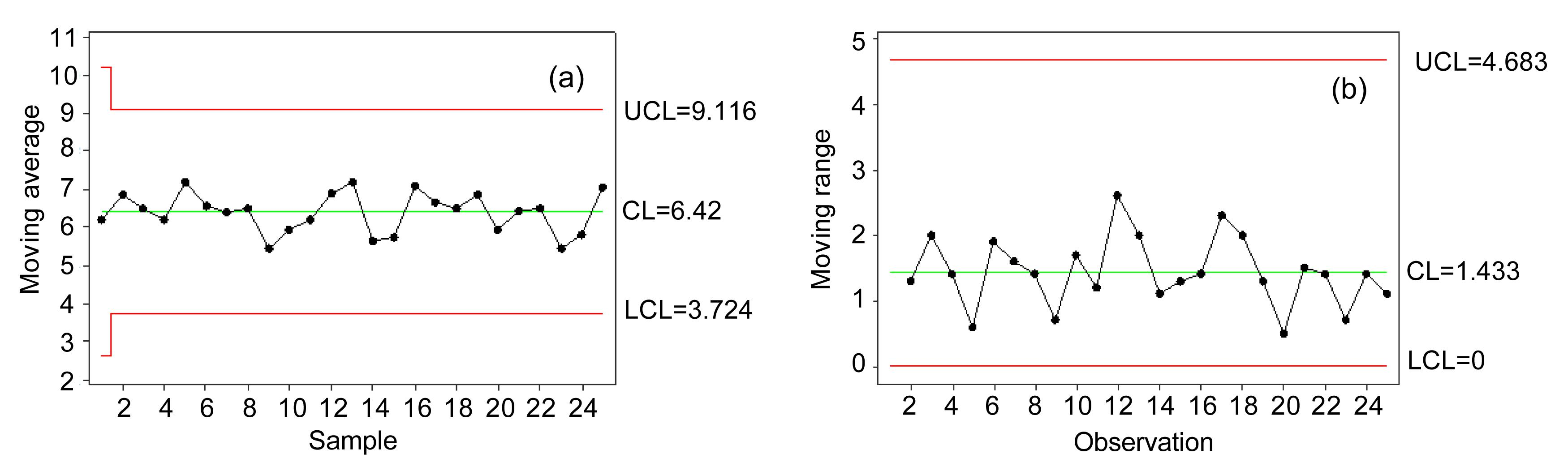Fig.8
MA control chart (a) and MR chart (b) for turbidity at optimal factor settings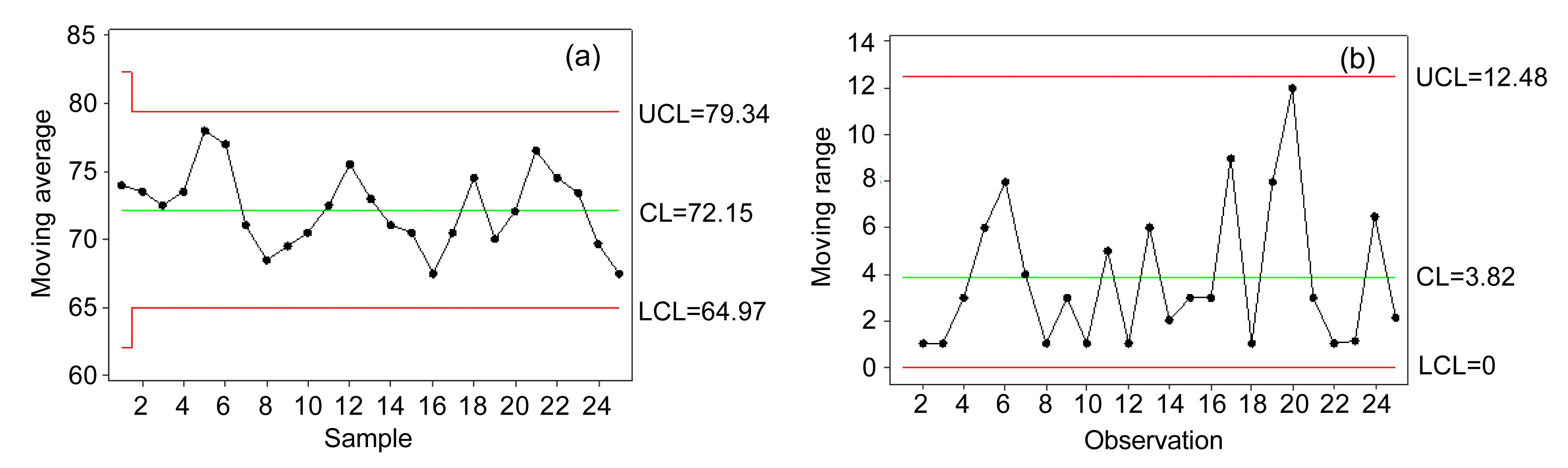Fig.9
MA control chart (a) and MR chart (b) for SVI at optimal factor settings

## 3.  Improvement analysis

The summary of the control chart results are displayed in Table 5. Process capability analysis will be utilized to measure the performance of the wastewater treatment process before and after the PAT framework. The most widely used process capability index is . However, this ratio does not take into account where the process mean is located relative to specifications. As a consequence, a new process capability ratio, , which takes process centering into account, will be used for measuring performance. is a function of and which are the one-sided process capability ratios. is expressed as follows: , where , , where and are the estimated process mean and standard deviation, respectively. Furthermore, the multiple capability index, MCpk, for a complete process of Q responses considered concurrently is calculated as .

#### Table 5

Summary of results from control charts
 Response MA MR UCL CL LCL UCL CL LCL y 1 Before 24.81 20.22 15.64 7.964 2.438 0.0 After 9.116 6.42 3.724 4.683 1.433 0.0 y 2 Before 92.69 79.92 67.15 22.19 6.79 0.0 After 79.34 72.15 64.97 12.48 3.82 0.0

The values for turbidity and SVI are compared at the initial and optimal factor settings, and then the results and improvement analysis are displayed in Table 6.

#### Table 6

Improvement analysis*
 Response LSL USL MCpk y 1 0 30 20.22 (6.42) 2.16 (1.27) 1.34 (5.5) 1.95 (10.6) y 2 50 100 79.69 (72.15) 6.02 (3.39) 1.46 (1.93)

• *Values at optimal settings are in parentheses

•  From Table 6 it is noted that:

1. For turbidity and SVI, the estimated process means at initial (optimal) factor settings are 20.22 (6.42) NTU and 79.69 (72.15) ml/g, respectively. The process means at optimal factor settings are improved significantly as they are closer to the desired values of less than 15 NTU and 70 ml/g, respectively.

2. The process standard deviations at initial (optimal) factor settings are 2.16 (1.27) NTU and 6.02 (3.39) ml/g for turbidity and SVI, respectively. Clearly, optimal factor settings results in significant variability reductions are 41.22% and 77.75% for turbidity and SVI, respectively.

3. The values are improved significantly due to setting factor levels at optimal settings. That is, the value for turbidity is improved from 1.34 to 5.5, while the value for SVI is enhanced from 1.46 to 1.93. These results indicate that the wastewater treatment process becomes highly efficient for both responses due to setting the factor at optimal levels.

4. The MCpk is improved significantly from 1.95 to 10.6, which also indicates that the process become highly capable.

## 4.  Conclusions

The PAT framework is successfully implemented to improve the performance of the wastewater treatment process for two main quality characteristics: turbidity and SVI. In this framework, the MA and MR control charts are used to assess the performance of the process at initial factor settings, where the control charts indicate in-control states for both responses. The 33 full factorial design is used for conducting experimental work. The weighted additive model is proposed, and then used for optimizing process performance while considering engineers’ preferences for factor settings and quality responses. Each response and process factor is described by suitable MF. The corresponding goal constraints are then defined. The objective function is used to minimize the weighted sum of the positive and negative deviations. Results show that the optimal process conditions found are flocculant dose of 18 mg/L, coagulant dose of 40.0 mg/L, and pH of 4.0. These parameters yield optimal values of turbidity and SVI of 6.184 NTU and 73.21 ml/g, respectively. Confirmation experiments are conducted at optimal factor settings. It is found that: (1) the process means for turbidity and SVI at optimal factor settings are closer to the desired values of less than 15 NTU and 70 ml/g, respectively; (2) process variability is significantly reduced by 41.22% and 77.75% for turbidity and SVI, respectively, and the MCpk is improved significantly from 1.95 to 10.6, which indicates that the process become highly capable. In conclusion, the weighted additive model is found to be an efficient technique for optimizing the performance of processes with multiple responses, taking into consideration the engineers’ preferences about process settings.

## ReferencesAl-Refaie, A., 2009. Optimizing SMT performance using comparisons of efficiency between different systems technique in DEA. IEEE Transactions on Electronics Packaging Manufacturing, 32(4):256-264.Al-Refaie, A., 2010. A grey-DEA approach for solving the multi-response problem in Taguchi method. Journal of Engineering Manufacture, 224(1):147-158.Al-Refaie, A., 2011. Optimizing correlated QCHs using principal components analysis and DEA techniques. Production Planning & Control, 22(7):676-689.Al-Refaie, A., 2012. Optimizing performance with multiple responses using cross-evaluation and aggressive formulation in DEA. IIE Transactions, 44(4):262-276.Al-Refaie, A., Li, M.H., 2011. Optimizing the performance of plastic injection molding using weighted additive model in goal programming. International Journal of Fuzzy System Applications, 1(2):43-54.Al-Refaie, A., Al-Tahat, M., 2011. Solving the multi-response problem in Taguchi method by benevolent formulation in DEA. Journal of intelligent Manufacturing, 22(4):505-521.Al-Refaie, A., Li, M.H., Tai, K.C., 2008. Optimizing SUS 304 wire drawing process by grey analysis utilizing Taguchi method. Journal of University of Science and Technology Beijing, Mineral, Metallurgy, Material, 15(6):714-722.Al-Refaie, A., Wu, T.H., Li, M.H., 2009. DEA approaches for solving the multi-response problem in Taguchi method. Artificial Intelligence for Engineering Design, Analysis and Manufacturing, 23(2):159-173.Al-Refaie, A., Rawabdeh, I., Alhajj, R., 2012. A fuzzy multiple-regression approach for optimizing multiple responces in the Taguchi method. International Journal of Fuzzy System Applications, 2(3):13-34.CDER (Center for Drug Evaluation and Research), 2004. Guidance for Industry: PAT-A Framework for Innovative Pharmaceutical Development, Manufacturing, and Quality Assurance, US Department of Health and Human Services Food and Drug Administration,:Muhammad, N., Manurng, Y.H., Hafidzi, M., 2012. Optimization and modeling of spot welding parameters with simultaneous multiple response consideration using multi-objective Taguchi method and RSM. Journal of Mechanical Science and Technology, 26(8):2365-2370.Salmasnia, A., Kazemzadeh, R.B., Tabrizi, M.M., 2012. A novel approach for optimization of correlated multiple responses based on desirability function and fuzzy logics. Neurocomputing, 91:56-66.Wang, J.P., Chen, Y.Z., Ge, X.W., 2007. Optimization of coagulation-flocculation process for a paper-recycling wastewater treatment using response surface methodology. Colloids and Surfaces A: Physicochemical and Engineering Aspects, 302(1-3):204-210.Wang, J.P., Chen, Y.Z., Ge, X.W., 2011. Optimization of coagulation-flocculation process for pulp mill wastewater treatment using a combination of uniform design and response surface methodology. Water Research, 45(17):5633-5640.Yaghoobi, M.A., Jones, D.F., Tamiz, M., 2008. Weighted additive models for solving fuzzy goal programming problems. Asia-Pacific Journal of Operational Research, 25(5):715-733.Ycel, A., Guneri, A.F., 2011. A weighted additive fuzzy programming approach for multi-criteria supplier selection. Expert Systems with Applications, 38(5):6281-6286.